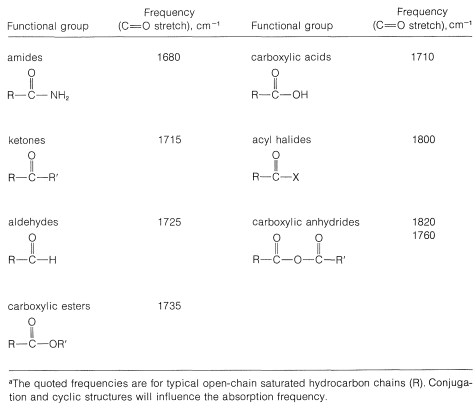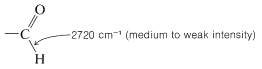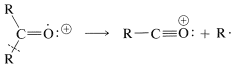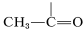# 16.4: Spectroscopic Properties

$$\newcommand{\vecs}{\overset { \rightharpoonup} {\mathbf{#1}} }$$ $$\newcommand{\vecd}{\overset{-\!-\!\rightharpoonup}{\vphantom{a}\smash {#1}}}$$$$\newcommand{\id}{\mathrm{id}}$$ $$\newcommand{\Span}{\mathrm{span}}$$ $$\newcommand{\kernel}{\mathrm{null}\,}$$ $$\newcommand{\range}{\mathrm{range}\,}$$ $$\newcommand{\RealPart}{\mathrm{Re}}$$ $$\newcommand{\ImaginaryPart}{\mathrm{Im}}$$ $$\newcommand{\Argument}{\mathrm{Arg}}$$ $$\newcommand{\norm}{\| #1 \|}$$ $$\newcommand{\inner}{\langle #1, #2 \rangle}$$ $$\newcommand{\Span}{\mathrm{span}}$$ $$\newcommand{\id}{\mathrm{id}}$$ $$\newcommand{\Span}{\mathrm{span}}$$ $$\newcommand{\kernel}{\mathrm{null}\,}$$ $$\newcommand{\range}{\mathrm{range}\,}$$ $$\newcommand{\RealPart}{\mathrm{Re}}$$ $$\newcommand{\ImaginaryPart}{\mathrm{Im}}$$ $$\newcommand{\Argument}{\mathrm{Arg}}$$ $$\newcommand{\norm}{\| #1 \|}$$ $$\newcommand{\inner}{\langle #1, #2 \rangle}$$ $$\newcommand{\Span}{\mathrm{span}}$$$$\newcommand{\AA}{\unicode[.8,0]{x212B}}$$

## Infrared Spectra

A carbonyl group in a compound can be positively identified by the strong infrared absorption band in the region $$1650$$-$$1850 \: \text{cm}^{-1}$$, which corresponds to the stretching vibration of the carbon-oxygen double bond. The position of the band within this frequency range depends on the molecular environment of the carbonyl group. As a result, we frequently can tell from the band position whether the structure is an aldehyde, ketone, carboxylic acid, ester, amide, or anhydride. The date of Table 16-3 show typical infrared absorption frequencies for specific types of carbonyl compounds. Thus aldehydes and ketones absorb at slightly lower frequencies (longer wavelengths) than carboxylic esters and anhydrides. We usually find that absorption shifts to lower frequencies $$\left( \sim 20 \: \text{cm}^{-1} \right)$$ when the carbonyl group is conjugated with other multiple bonds, as in aromatic ketones, $$\ce{C_6H_5COCH_3}$$.

Table 16-3: Characteristic Infrared Absorption Frequencies of Carbonyl Compounds$$^a$$Aldehydes can be distinguished from ketones by a band at $$2720 \: \text{cm}^{-1}$$ which is characteristic of the $$\ce{C-H}$$ stretching vibration of an aldehyde function:This band is unusually low in frequency for a $$\ce{C-H}$$ stretching vibration; although the band is rather weak, it occurs in a region of the spectrum where other absorptions generally are absent so it can be identified with no special difficulty.

## Electronic Absorption Spectra

Aldehydes and ketones absorb ultraviolet light in the region $$275$$-$$295 \: \text{nm}$$, and the result is excitation of an unshared electron on oxygen to a higher energy level. This is the $$n \rightarrow \pi^*$$ transition discussed in Section 9-9. A more intense $$\pi \rightarrow \pi^*$$ transition occurs about \180\)-$$190 \: \text{nm}$$, which corresponds to excitation of an electron from a $$\pi$$-bonding orbital to a $$\pi$$-antibonding orbital. Neither of these absorptions is especially useful for specific identification unless the carbonyl group is conjugated, in which case the $$n \rightarrow \pi^*$$ and $$\pi \rightarrow \pi%+^*$$ bands occur at longer wavelengths (by $$30$$-$$40 \: \text{nm}$$). For example, if you suspect that a compound is an alkenone from its infrared spectrum, you easily could tell from the $$\lambda_\text{max}$$ of the $$n \rightarrow \pi^*$$ and $$\pi \rightarrow \pi^*$$ absorptions of the compound whether it is a conjugated alkenone. The absorption frequency would be expected around $$320 \: \text{nm}$$ and $$220 \: \text{nm}$$ (see Figure 9-20).

## Mass Spectra

Aldehydes and ketones generally give moderately intense signals due to their molecular ions, $$\ce{M^+}$$. Thus the determination of the molecular weight of a ketone by mass spectroscopy usually is not difficult. Furthermore, there are some characteristic fragmentation patterns that aid in structural identification. These are:

• $$\alpha$$ cleavage• transfer of $$\gamma$$ hydrogen with $$\beta$$ cleavage (McLafferty rearrangement)## NMR Spectra

The character of the carbonyl bond gives rise to very low-field nmr absorptions for the proton of an aldehyde group $$\left( \ce{-CH=O} \right)$$. As Table 9-4 shows, these absorptions are some $$4 \: \text{ppm}$$ to lower fields than alkenyl hydrogens (). Some of this difference in shift can be ascribed to the polarity of the carbonyl group $$\overset{\delta \oplus}{\ce{C}} \ce{=} \overset{\delta \ominus}{\ce{O}}$$, which reduces electron density around the aldehyde hydrogen (see Section 9-10E). The effect appears to carry over in much smaller degree in the $$\alpha$$ positions, and protons of the typeare about $$0.3 \: \text{ppm}$$ to lower fields than those of.